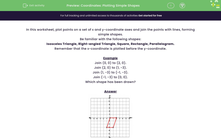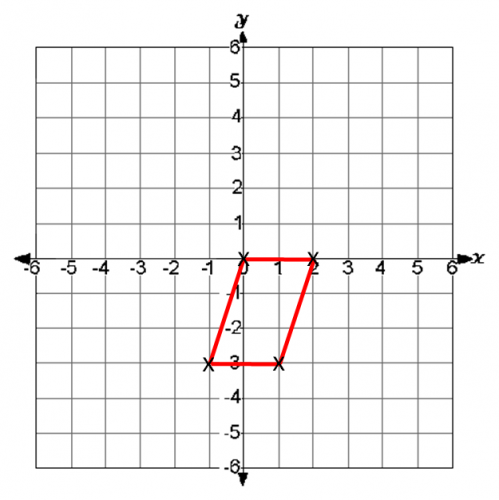# Coordinates: Plotting Simple Shapes

In this worksheet, students must plot points on a set of x-y coordinate axes and join certain points to form simple 2D shapes.Key stage:  KS 2

Curriculum topic:   Geometry: Position and Direction

Curriculum subtopic:   Draw/Translate Shapes

Popular topics:   Geometry worksheets

Difficulty level:#### Worksheet Overview

In this worksheet, plot points on a set of x and y-coordinate axes and join the points with lines, forming simple shapes.

Be familiar with the following shapes:

Isosceles Triangle, Right-angled Triangle, Square, Rectangle, Parallelogram.

Remember that the x-coordinate is plotted before the y-coordinate.

Example

Join (0, 0) to (2, 0).

Join (2, 0) to (1, -3).

Join (1, -3) to (-1, -3).

Join (-1, -3) to (0, 0).

Which shape has been drawn?We have drawn a Parallelogram.

### What is EdPlace?

We're your National Curriculum aligned online education content provider helping each child succeed in English, maths and science from year 1 to GCSE. With an EdPlace account you’ll be able to track and measure progress, helping each child achieve their best. We build confidence and attainment by personalising each child’s learning at a level that suits them.

Get started